Math Calculators, Lessons and Formulas

It is time to solve your math problem

mathportal.org
• Quadratic Equations, Inequalities, and Functions

ans:
syntax error
C
DEL
ANS
±
(
)
÷
×
7
8
9
4
5
6
+
1
2
3
=
0
.
auto next question
calculator
•  Question 1: 1 pts The equation of quadratic function which graph is shown in the figure at the right is: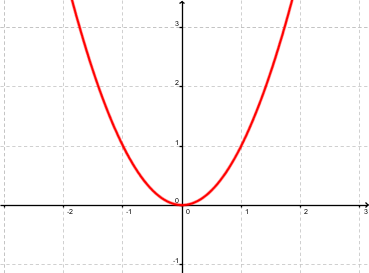$f(x)=-x^2$ $f(x)=x^2$ $f(x)=-{(x+1)}^2$ $f(x)={(x+1)}^2$
•  Question 2: 1 pts The equation of quadratic function which graph is shown in the figure at the right is: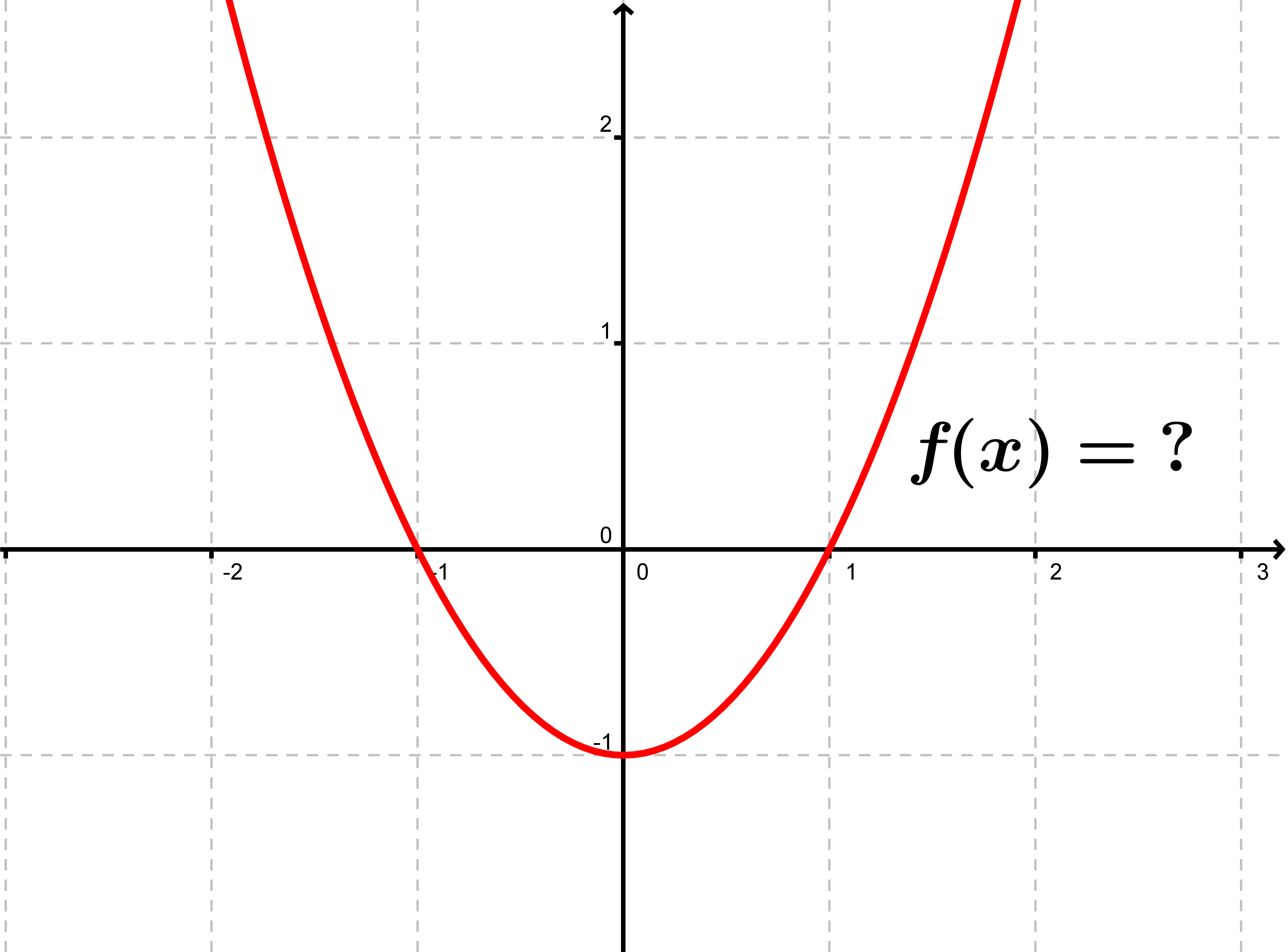$f(x)=x^2+1$ $f(x)=x^2-1$ $f(x)=-x^2+1$ $f(x)=-x^2-1$
•  Question 3: 1 pts The graph of quadratic function $f(x)=-21x^2+14x-23$ opens downward.
•  Question 4: 2 pts Graphs of functions $f(x) = x^2$ and $g(x)={\frac12}x^2$ are shown at the right. Which of the following is correct?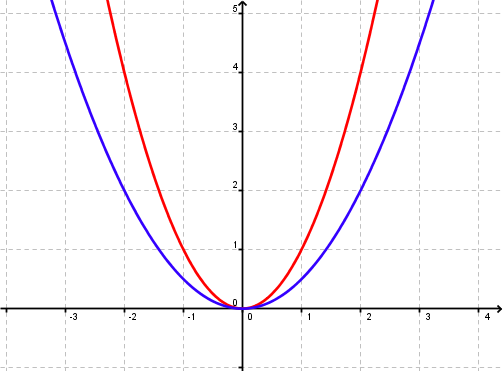blue line is a graph of $f(x)$ blue line is a graph of $g(x)$
•  Question 5: 2 pts Graphs of functions $f(x)=0.23x^2-1.3x$ and $g(x)=-0.53x^2+2x$ are shown at the right. Which of the following is correct?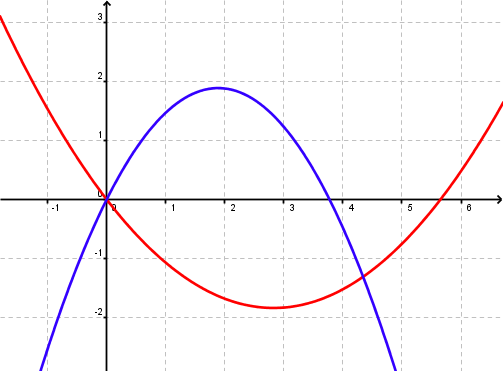blue line is a graph of $f(x)$ blue line is a graph of $g(x)$
•  Question 6: 2 pts Which of the following graphs represent $f(x)=x^2-3x$ ?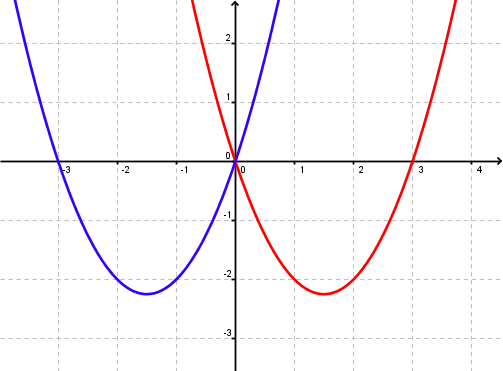blue graph red graph
•  Question 7: 2 pts Consider a graph of the function $f(x)=ax^2+bx+c$ shown at the right. Which of the following statements is true?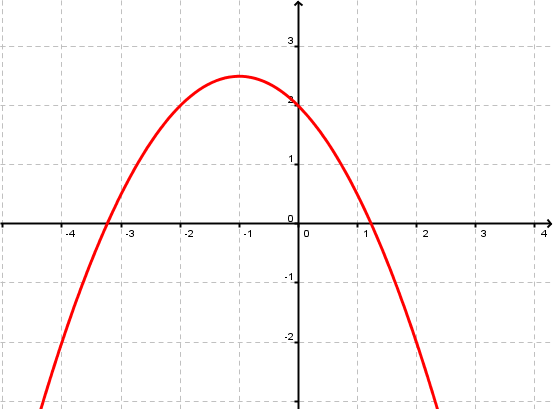$a>0$ $a<0$ the sign of $a$ cannot be determined
•  Question 8: 2 pts Consider a graph of the function $f(x)=ax^2+bx+c$ shown at the right. Which of the following statements is true?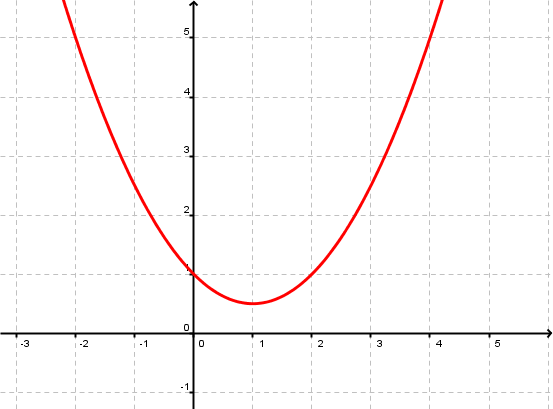$a>0$ $a<0$ the sign of $a$ cannot be determined
•  Question 9: 3 pts Consider a graph of the function $f(x)=ax^2+bx+c$ shown at the right. Which of the following statements is true?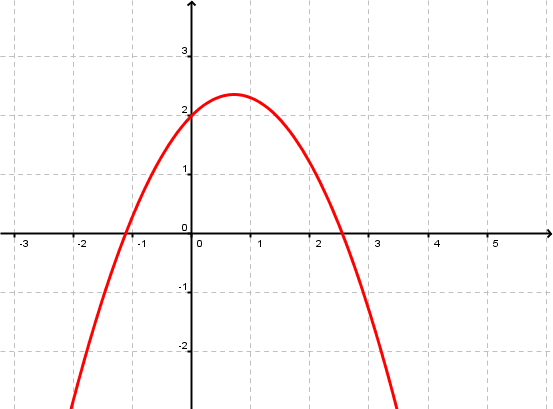$a>0$ $c>0$ $a>0$ $c<0$ $a<0$ $c>0$ $a<0$ $c<0$
•  Question 10: 3 pts Consider a graph of the function $f(x)=ax^2+bx+c$ shown at the right. Which of the following statements is true?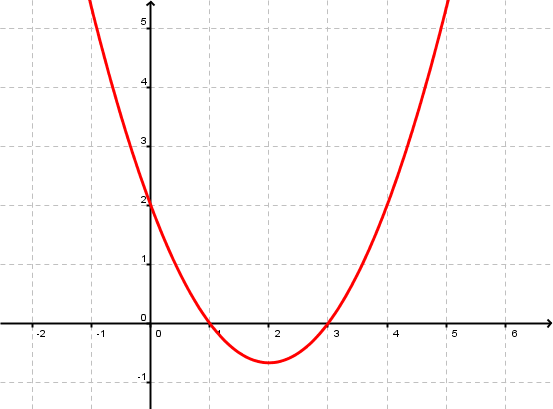$a<0$ $c=2$ $a>0$ $c=2$ $a<0$ $c=1$ $a>0$ $c=1$
•  Question 11: 3 pts Which of the following graphs represent $f(x)={\frac12}x^2-2x+1$ ?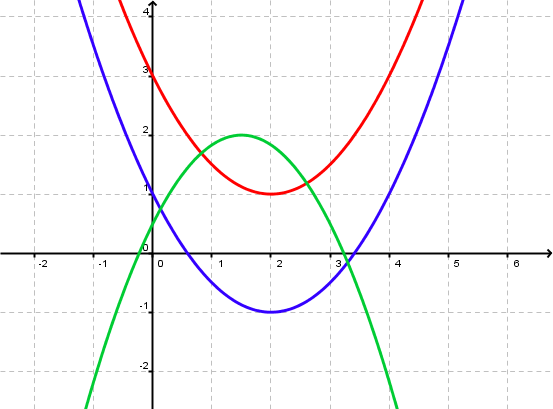red graph blue graph green graph
•  Question 12: 3 pts Which of the following graphs represent $f(x)=-{(x+2)}^2$ ?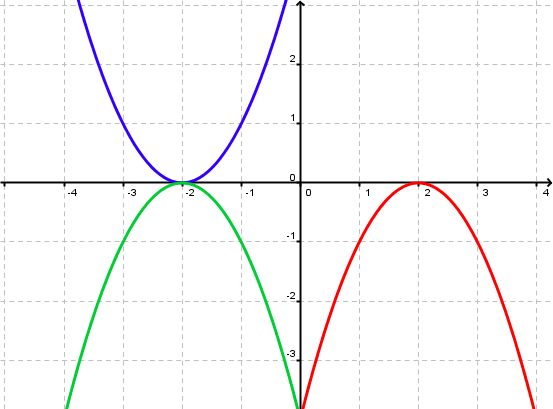red graph blue graph green graph
•  Question 13: 3 pts What is the equation of the function with graph at the left?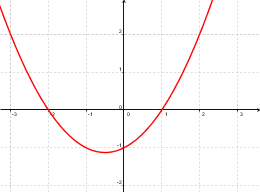$f(x)=(x-1)(x+2)$ $f(x)=(x+1)(x-2)$ $f(x)=1/2(x-1)(x+2)$ $f(x)=1/2(x+1)(x-2)$